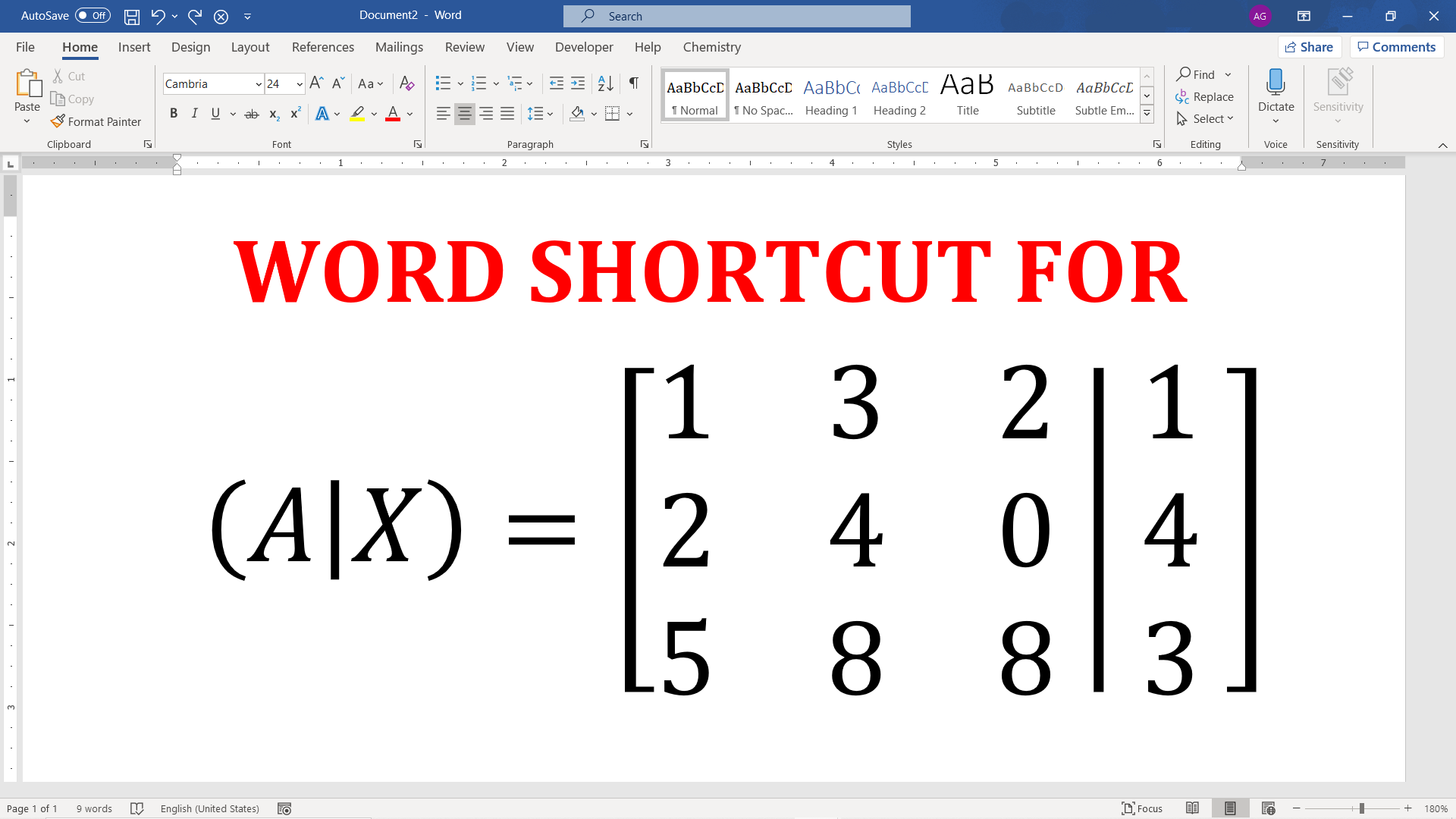# How to type Augmented Matrix in Word?Augmented matrix appears at many places in linear algebra. It is useful to visualize how matrix changes upon similar row operations. It is created by appending column of one matrix to another with a vertical line as a matrix separator.

Following matrix is an example of an augmented matrix.## Augmented matrix in Microsoft Word

The important step is to get the augmented matrix brackets and separator (|) that can expand to the height of the matrix.

The entire procedure to insert augmented matrix in Word is show in the following video.

## Steps to get augmented matrix in Word

• Get equation editor box at the location where you want to get augmented matrix.
• Method 1: Insert equation editor by navigating Insert Tab > Equations or,
• Method 2: Use Alt + =Shortcut to insert equation editor.
• Inside equation editor type [|] and hit space immediately after it. This gives a place holders for matrix with expandable brackets and matrix separator.